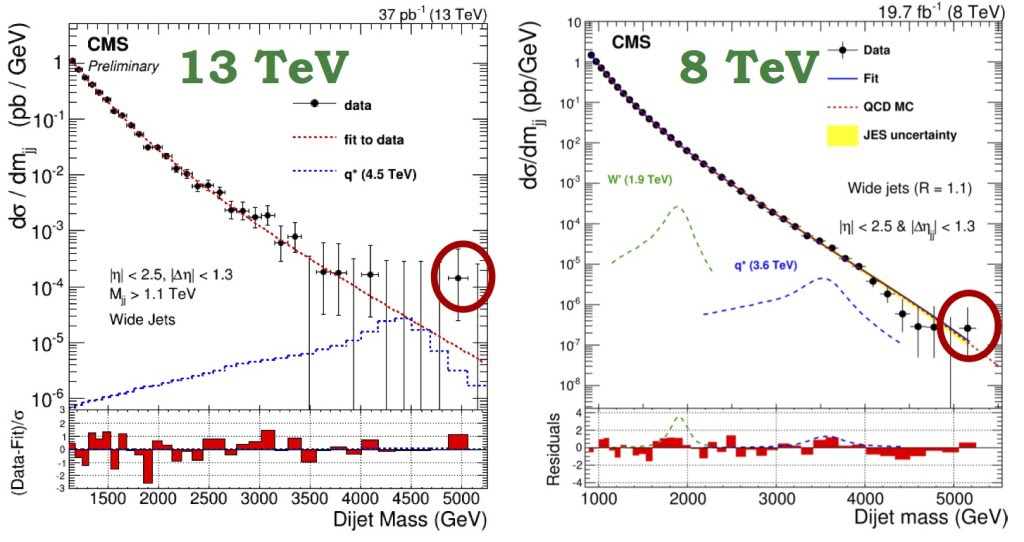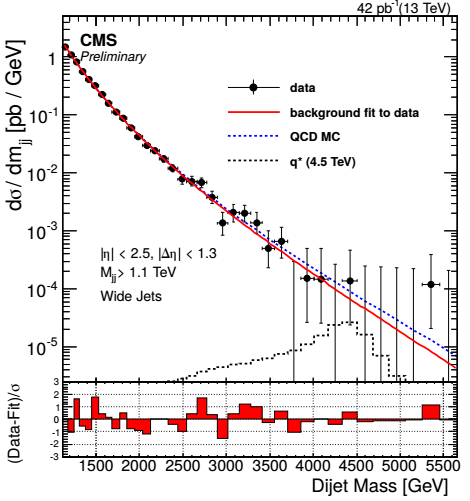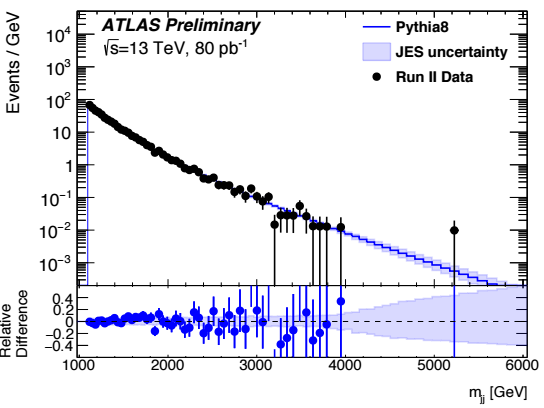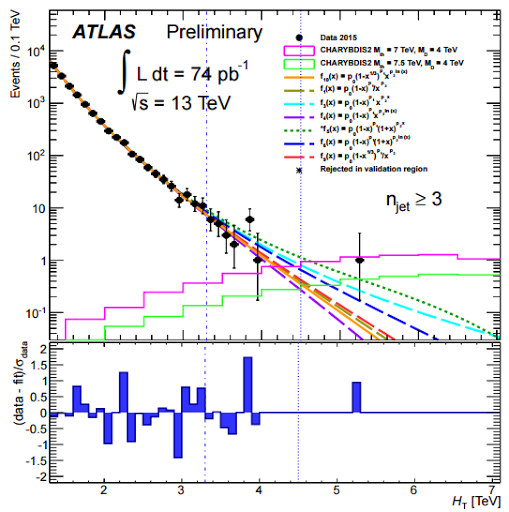Tuesday, September 01, 2015

Both CMS and ATLAS see $$5.2\TeV$$ dijet (and trijet)

One month ago, I discussed two intriguing dijet events seen by the CMS collaboration at the LHC. They had a pretty high mass. An event from the $$8\TeV$$ run in 2012 had the total mass of $$5.15\TeV$$ while a fresh event from the $$13\TeV$$ run in 2015 had the total mass of $$5.0\TeV$$ or so.

Two collisions isn't a terribly high number so these events, even though their energy is really higher than the energy of the "following" high-energy events, may deserve to be ignored even more strictly than the bumps in many other searches.

Perhaps, to make you more excited, you were saying, the competitors at ATLAS would have to see the $$5.2\TeV$$ event as well. Moreover, a miracle should better cut the $$0.2\TeV$$ gap between the two CMS events. What happened a month later?

Ladies and Gentlemen, the competitors at ATLAS have seen the $$5.2\TeV$$ event as well. Moreover, CMS has adjusted their energies and the $$5.0\TeV$$ event from the 2015 run is quoted as a $$5.2\TeV$$ event as well so the gap is gone! And as a bonus, ATLAS has also seen a $$5.2\TeV$$ event in a multijet analysis. Isn't it starting to look intriguing?

Last night, ATLAS released three papers from the $$13\TeV$$ run while the CMS showed us one. ATLAS gives us an analysis of Z-plus-jets, both teams unmask their new narrow dijet analyses, and ATLAS adds a search for strong gravity with trijets and similar beasts.

At the end of July, you were given this picture from CMS:The right graph shows the highest energy dijet from the $$8\TeV$$ run to be $$5.2\TeV$$ but it doesn't look impressive because there are other nearby high-energy dijets, too. However, the left graph from the fresh $$13\TeV$$ run shows a rather big gap followed by one $$5.0\TeV$$ event. They could be signs of the same particle.

Now, CMS has provided us with the paper which contains a new version of this left graph:The total luminosity is 42 inverse picobarns instead of 37 – not too much progress in the last month – but you see that the graph is basically the same except that the estimated energy of the dijets has been significantly increased and now the black swan event has $$5.2\TeV$$ or $$5.3\TeV$$.

What about ATLAS? They have a dijet search out, too. Figure 13 shows a colorful "photograph" of their highest-mass event in the dijet mass search. The scalar sum of the jet $$p_T$$ is $$5.2\TeV$$. The ATLAS search has found "nothing conclusive" but Figures 1,3,7 are pretty cool for our discussion. Here is Figure 7:Just look how isolated the $$5.2\TeV$$ ATLAS dijet event seems to be. In every $$100\GeV$$ wide bin up to $$4.0\TeV$$ except for the bin between $$3.8$$ and $$3.9\TeV$$, there is at least one event. Then there is a big window $$4.0$$-$$5.2\TeV$$ with no events at all. And then you have the isolated collision in $$5.2$$-$$5.3\TeV$$, the same bin as one in the new CMS analysis.

Note that these $$5.2\TeV$$ events were two totally different collisions that took place at different places – inside the ATLAS detector and the CMS detector, respectively. CMS is a more compact but chunky detector in France. ATLAS is a larger but relatively lighter one on the opposite side of the ring. Because it's in Switzerland, as the Swiss anthem at 0:12 of Don Garbutt's video above proves, the ATLAS collisions didn't even take place in the European Union. ;-) But these collisions seem to speak the same language (namely French LOL).

And there's a bonus. ATLAS has also presented its search for strong gravity. No conclusive evidence of anything has been seen but look at this Figure 7:It shows events with at least three jets – so the dijet event we mentioned previously should be absent. The graph shows lots of events up to $$4\TeV$$, then a big desert, and then an event between $$5.2$$ and $$5.3\TeV$$. Well, the $$x$$-axis isn't really the mass in this case, it's the $$H_T$$ variable – the sum of $$p_T$$ of three or four leading jets, of the lepton if any, and of the missing transverse energy. But for such extremely high-energy collisions, $$H_T$$ and $$m$$ could be very close, my intuition says.

So right now, we seem to have four collisions in four different searches – two from CMS and two from ATLAS – that seem to contain a hint of a particle of mass $$5.2\TeV$$ capable of decaying to jets. Well, the number of these $$5.2\TeV$$ events should better double again. Wait for another month. ;-)